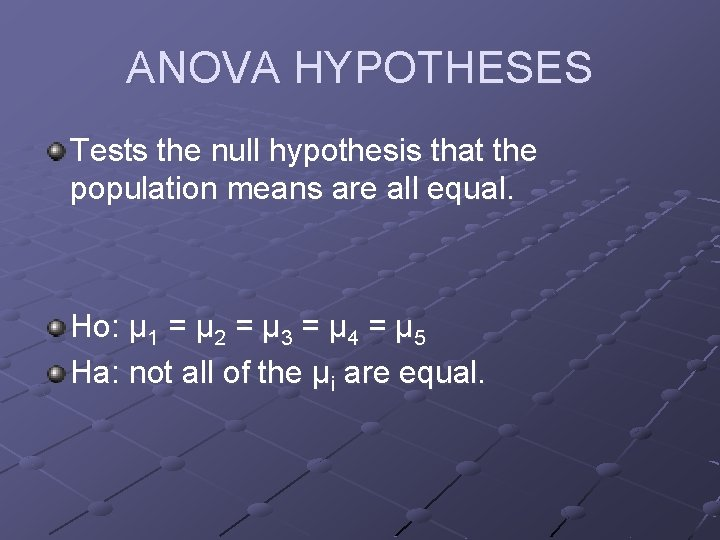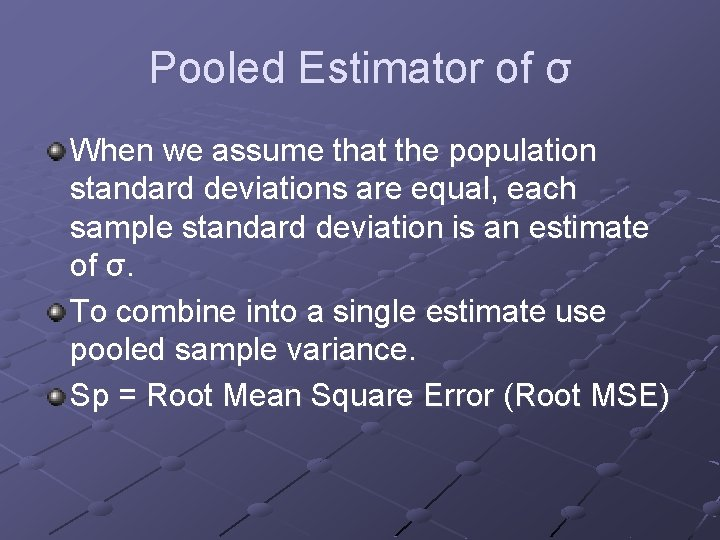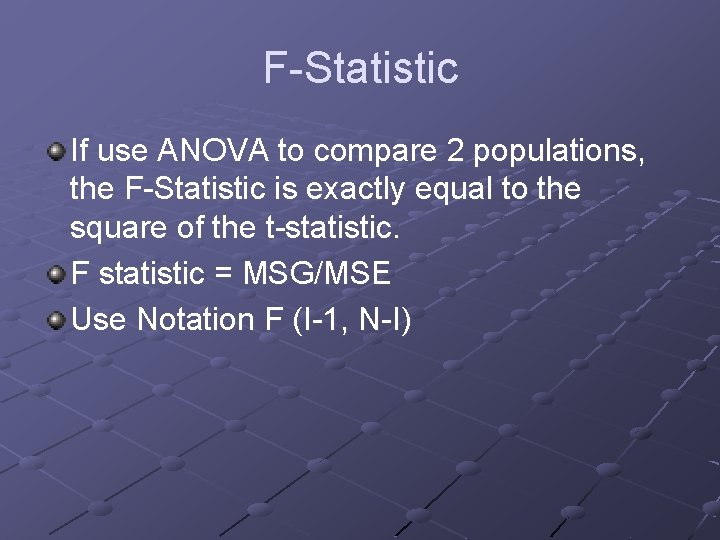# ANOVA One Way Analysis of Variance ANOVA Purpose

• Slides: 8ANOVA One Way Analysis of VarianceANOVA Purpose: To assess whethere are differences between means of multiple groups. ANOVA provides evidence. We compare variation among the means of the groups with the variation within groups. Hence the name Analysis of VarianceANOVA HYPOTHESES Tests the null hypothesis that the population means are all equal. Ho: μ 1 = μ 2 = μ 3 = μ 4 = μ 5 Ha: not all of the μi are equal.ANOVA ASSUMPTIONS The populations are normally distributed. The standard deviations of the populations are equal. Moore and Mc. Cabe p. 755: n Rule for examining standard deviation in ANOVA n If the largest standard deviation is less than twice the smallest standard deviation, we can use methods based on the assumption of equal standard deviations and our results will be approximately correct.Pooled Estimator of σ When we assume that the population standard deviations are equal, each sample standard deviation is an estimate of σ. To combine into a single estimate use pooled sample variance. Sp = Root Mean Square Error (Root MSE)ANOVA TABLE Sum of Squares—represents variation in data, a sum of squared deviations SSTotal = SSGroups + SSError SST - Measures variation around the overall mean SSG-Variation of the groups means around the overall means SSE-measures variation of each observation around its group mean.ANOVA Table Continued Degrees of Freedom n n n Degrees of Freedom Total = N – 1 Degrees of Freedom Group = I – 1 Degrees of Freedom Error = N – I (I = number of groups) DFT = DFG + DFE Sp 2 = MSE = SSE/DFE MSG = SSG/DFGF-Statistic If use ANOVA to compare 2 populations, the F-Statistic is exactly equal to the square of the t-statistic. F statistic = MSG/MSE Use Notation F (I-1, N-I)